JMSLTM Numerical Library 7.2.0
com.imsl.stat

Class ARMAEstimateMissing

• All Implemented Interfaces:
Serializable

public class ARMAEstimateMissing
extends Object
implements Serializable
Estimates missing values in a time series collected with equal spacing. Missing values can be replaced by these estimates prior to fitting a time series using the ARMA class.

Traditional time series analysis as described by Box, Jenkins and Reinsel (1994) requires the observations be made at equidistant time points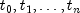where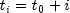. When observations are missing, ARMA requires that they be replaced with suitable estimates. Class ARMAEstimateMissing offers 4 methods for estimating missing values: MEDIAN, CUBIC_SPLINE, AR_1, and AR_P

Method MEDIAN estimates the missing observations in a gap by the median of the last four time series values before and the first four values after the gap. If not enough values are available before or after the gap then the number is reduced accordingly. This method is very fast and simple, but its use is limited to stationary ergodic series without outliers and level shifts.

Method CUBIC_SPLINE uses a cubic spline interpolation method to estimate missing values. Here the interpolation is again done over the last four time series values before and the first four values after the gap. The missing values are estimated by the resulting interpolant. This method gives smooth transitions across missing values.

Method AR_1 assumes that the time series before the gap can be approximated using an AR(1) process. If the last observation prior to the gap is made at time point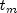then this method uses values at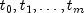to compute the one-step-ahead forecast at origin. This value is used to estimate the missing value at time point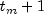. If the value at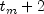is also missing then the values at time points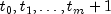are used to recompute the AR(1) model, and then estimate the value atand so on. The coefficient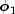in the AR(1) model is computed internally by the method of least squares from class ARMA.

Finally, method AR_P uses an AR(p) model to estimate missing values using a one-step-ahead forecast similar to method AR_1. First, class ARAutoUnivariate, is applied to the time series values just prior to the missing values to determine the optimum p from the set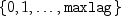of possible values and to compute the parameters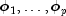of the resulting AR(p) model. The parameters are estimated by the least squares method based on Householder transformations as described in Kitagawa and Akaike (1978). Denoting the mean of the series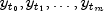by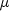the one-step-ahead forecast at origin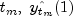, can be computed by the formula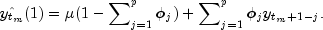This value is used as an estimate for the missing value at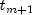. The procedure starting with ARAutoUnivariate is then repeated for every further missing value in the gap. All four estimation methods treat gaps of missing values in increasing time order.

Example, Serialized Form
• Field Summary

Fields
Modifier and Type Field and Description
static int AR_1
Indicates that missing values should be estimated using an autoregressive time series with 1 lag.
static int AR_P
Indicates that missing values should be estimated using an autoregressive time series with a maximum lag of maxLag.
static int CUBIC_SPLINE
Indicates that missing values should be estimated using cublic spline interpolation.
static int LEAST_SQUARES
Estimate autoregressive coefficients using least squares.
static int MAX_LIKELIHOOD
Estimate autoregressive coefficients using maximum likelihood.
static int MEDIAN
Indicates that missing values should be estimated using the median of the values just before and after the missing value gap.
static int METHOD_OF_MOMENTS
Estimate autoregressive coefficients using method of moments.
• Constructor Summary

Constructors
Constructor and Description
ARMAEstimateMissing(int[] tpoints, double[] z)
Constructor for ARMAEstimateMissing.
• Method Summary

Methods
Modifier and Type Method and Description
int[] getCompleteTimes()
Returns an int array of all time points, including values for times with missing values in z.
double[] getCompleteTimeSeries()
Returns a double precision vector of length tpoints[tpoints.length-1]-tpoints+1 containing the observed values in the time series z plus estimates for missing values in gaps identified in tpoints.
double getConvergenceTolerance()
Returns the current value of convergence tolerance used by the AR_1 and AR_P estimation methods.
int getEstimationMethod()
Returns the method used for estimating the final autoregressive coefficients for missing value estimation methods AR_1 and AR_P.
int getMaxIterations()
Returns the maximum number of estimation iterations used by missing value estimation methods AR_1 and AR_P.
int getMaxlag()
Returns the current value of autoregressive lags used in the AR_P estimation method.
double getMean()
Returns the mean value used to center the series.
int[] getMissingTimes()
Returns an int array of the times with missing values.
int getMissingValueMethod()
Returns the current missing value estimation method.
int getNumberMissing()
Returns the number of missing values in the original series
double getRelativeError()
Returns the relative error used for the METHOD_OF_MOMENTS and LEAST_SQARES estimation methods.
void setConvergenceTolerance(double convergenceTolerance)
Sets the covergence tolerance used by the AR_1 and AR_P missing value estimation methods.
void setEstimationMethod(int arEstimationMethod)
Sets the method used for estimating the autoregressive coefficients for missing value estimation methods AR_1 and AR_P.
void setMaxIterations(int maxIterations)
Sets the maximum number of estimation iterations for missing value estimation methods AR_1 and AR_P.
void setMaxlag(int maxlag)
Sets the maximum number of autoregressive lags when method AR_P is selected as the missing value estimation method.
void setMean(double mean)
Sets the mean value used to center the series.
void setMissingValueMethod(int method)
Sets the current missing value estimation method to MEDIAN, CUBIC_SPLINE, AR_1, or AR_P.
void setRelativeError(double relativeError)
Sets the relative error used for the METHOD_OF_MOMENTS and LEAST_SQUARES estimation methods.
• Field Detail

• AR_1

public static final int AR_1
Indicates that missing values should be estimated using an autoregressive time series with 1 lag.
Constant Field Values
• AR_P

public static final int AR_P
Indicates that missing values should be estimated using an autoregressive time series with a maximum lag of maxLag. By default maxLag=10, but this can be changed using the setMaxlag method.
Constant Field Values
• CUBIC_SPLINE

public static final int CUBIC_SPLINE
Indicates that missing values should be estimated using cublic spline interpolation.
Constant Field Values
• LEAST_SQUARES

public static final int LEAST_SQUARES
Estimate autoregressive coefficients using least squares.
Constant Field Values
• MAX_LIKELIHOOD

public static final int MAX_LIKELIHOOD
Estimate autoregressive coefficients using maximum likelihood.
Constant Field Values
• MEDIAN

public static final int MEDIAN
Indicates that missing values should be estimated using the median of the values just before and after the missing value gap.
Constant Field Values
• METHOD_OF_MOMENTS

public static final int METHOD_OF_MOMENTS
Estimate autoregressive coefficients using method of moments.
Constant Field Values
• Constructor Detail

• ARMAEstimateMissing

public ARMAEstimateMissing(int[] tpoints,
double[] z)
Constructor for ARMAEstimateMissing.
Parameters:
tpoints - an int array containing the times at which the series values were observed. The values must be strictly increasing. Times for missing values are identified as non-incremental gaps in this series. A gap of missing values in z is assumed when the difference between two consecutive values is greater than 1, i.e.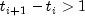. The difference is the number of missing values in the gap. The series can have multiple gaps with missing values, but any one gap can have no more than 3 missing values.
z - a double array containing the values for the time series observed at the times given in the vector tpoints.
• Method Detail

• getCompleteTimes

public int[] getCompleteTimes()
Returns an int array of all time points, including values for times with missing values in z.
Returns:
An int array of all times from tpoints=1 to tpoints.length+nMissing. Where nMissing is the number of values removed from the original time series, nMissing = getNumberMissing().
• getConvergenceTolerance

public double getConvergenceTolerance()
Returns the current value of convergence tolerance used by the AR_1 and AR_P estimation methods.
Returns:
a double scalar value equal to the convergence tolerance. By default the convergence tolerance is 1.0e-09.
• getEstimationMethod

public int getEstimationMethod()
Returns the method used for estimating the final autoregressive coefficients for missing value estimation methods AR_1 and AR_P.
Returns:
an int representing the estimation method used for estimating the autoregressive coefficients. 0 implies METHOD_OF_MOMENTS, 1 implies LEAST_SQUARES, 2 implies MAX_LIKELIHOOD.
• getMaxIterations

public int getMaxIterations()
Returns the maximum number of estimation iterations used by missing value estimation methods AR_1 and AR_P.
Returns:
An int scalar equal to the maximum number of iterations for the maximum likelihood missing value estimation method. If this limit is exceeded during the compute method, ARMAEstimateMissing stops execution and issues an ARMAMaxLikelihood.IterationLimitExceededException.
• getMaxlag

public int getMaxlag()
Returns the current value of autoregressive lags used in the AR_P estimation method.
Returns:
An int scalar value equal to the maximum number of autoregressive lags used with the AR_P missing value estimation method.
• getMean

public double getMean()
Returns the mean value used to center the series.
Returns:
a double scalar used to center the series.
• getMissingTimes

public int[] getMissingTimes()
Returns an int array of the times with missing values.
Returns:
An int array containing the times at which missing values were estimated. If there are no missing values a null array is returned.
• getMissingValueMethod

public int getMissingValueMethod()
Returns the current missing value estimation method.
Returns:
an int representing the estimation method used for estimating the missing values in the time series. 0 implies MEDIAN, 1 implies CUBIC_SPLINE, 2 implies AR_1 and 3 implies AR_P.
• getNumberMissing

public int getNumberMissing()
Returns the number of missing values in the original series
Returns:
An int scalar value containing the number of missing values in the time series.
• getRelativeError

public double getRelativeError()
Returns the relative error used for the METHOD_OF_MOMENTS and LEAST_SQARES estimation methods.
Returns:
a double scalar containing the stopping criterion for use in the nonlinear equation solver used in both the method of moments and least-squares algorithm.
• setConvergenceTolerance

public void setConvergenceTolerance(double convergenceTolerance)
Sets the covergence tolerance used by the AR_1 and AR_P missing value estimation methods.
Parameters:
convergenceTolerance - A double scalar value. Default: convergenceTolerance = 1.0e-09
• setEstimationMethod

public void setEstimationMethod(int arEstimationMethod)
Sets the method used for estimating the autoregressive coefficients for missing value estimation methods AR_1 and AR_P.
Parameters:
arEstimationMethod - An int scalar specifying the method used to estimate the autoregressive coefficients. Valid methods are METHOD_OF_MOMENTS, LEAST_SQUARES, and MAX_LIKELIHOOD. By default, arEstimationMethod=LEAST_SQUARES.
• setMaxIterations

public void setMaxIterations(int maxIterations)
Sets the maximum number of estimation iterations for missing value estimation methods AR_1 and AR_P. If this limit is exceeded ARMAEstimateMissing stops execution during the compute method and issues an IterationLimitExceededException.
Parameters:
maxIterations - An int specifying the maximum number of iterations for the maximum likelihood estimation. By default, maxIterations=200.
• setMaxlag

public void setMaxlag(int maxlag)
Sets the maximum number of autoregressive lags when method AR_P is selected as the missing value estimation method.
Parameters:
maxlag - An int scalar value equal to the maximum number of autoregressive lags. maxlag must be greater than z.length-5. By default maxlag=10.
• setMean

public void setMean(double mean)
Sets the mean value used to center the series.
Parameters:
mean - a double scalar used to center the series. By default the median of the series is used for centering.
• setMissingValueMethod

public void setMissingValueMethod(int method)
Sets the current missing value estimation method to MEDIAN, CUBIC_SPLINE, AR_1, or AR_P.
Parameters:
method - An int scalar. By default method=AR_1.
• setRelativeError

public void setRelativeError(double relativeError)
Sets the relative error used for the METHOD_OF_MOMENTS and LEAST_SQUARES estimation methods.
Parameters:
relativeError - a double scalar containing the stopping criterion for use in the nonlinear equation solver used in both the method of moments and least-squares algorithm. Default: relativeError = 2.22045e-14
JMSLTM Numerical Library 7.2.0